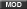Not a customer? Start a free trial

Click the Start a free trial link to start a 15-day SaaS trial of our product and join our community as a trial user. If you are an existing customer do not start a free trial.

AppDynamics customers and established members should click the sign in button to authenticate.

cancel
Showing results for
Show  only  | Search instead for
Did you mean:

## What Metric Transformers can I find in the extensions commons library?Moderator

# Metric Transformers in the Extensions Commons Library

The Java Extension SDK provides an automated utility that allows extensions developers to transform a metric name and value as it would be represented in the Metric Browser. These transformers include the Alias, the Multiplier, the Delta, and the Convert.

## How should the transformers be configured?

In the `metrics.xml`, the transformers should be specified in the following manner.

```   <stat url="/nitro/v1/stat/lbvserver" rootElement="lbvserver" alias="Load Balancing Server Metrics">
<metric attr="cursrvrconnections" alias="Server Connections"/>
<metric attr="memoryInMBytes" alias="Memory in KB" multiplier="1000"/>
<metric attr="throughput" delta="true" />
<metric attr="state">
<convert str="DOWN" value="0"/>
<convert str="UP" value="1"/>
<convert str="UNKNOWN" value="2"/>
</metric>
</stat>```

In the `config.yml`, the transformers should be specified in the following manner.

```metrics:
- name: “cursrvrconnections”
alias: “Cursor RV Connections”
delta: true
multiplier: 1000
convert:
“DOWN”: 0
“UP”: 1
“UNKNOWN”: 2```

Note that multiple transformers can be applied to the same metric as follows:

` <metric attr="cursrvrconnections" alias="Server Connections" multiplier="10" delta="true"/>`

1. It is not mandatory to specify any of these transformers.
2. By default, a metric does not have an alias and will be named as-is from the artifact being monitored.
3. The default value for the multiplier is always 1.
4. The default value for the delta is always false.
5. A metric that yields text values will simply be skipped if a convert transformer isn’t specified.

On calling `transformAndPrintMetrics(List<Metric> metrics)` in `com.appdynamics.extensions.MetricWriteHelper`, the SDK automatically applies any configured transformers before publishing these metrics.

## List of transformers

#### Alias

The Alias transformer can be used to replace a metric’s name. Often, metrics are represented by ambiguous names in the artifacts being monitored, as evident in the example above. In this case, cursrvrconnections will be represented as Server Connections in the Metric Browser.

#### Multiplier

The Multiplier transformer can be used to multiply the original metric value by a configured factor. From the example above, consider that an artifact returns memory in MB and a requirement is to monitor this metric in KB. A multiplier of 1000 can be applied to this metric to satisfy this requirement.

#### Delta

Consider an ever-increasing metric value and a requirement is to monitor the number of units by which this metric increases every minute. A Delta transformer can be applied in this case. This transformer compares the value of a metric at minute X with its value at minute X-1 and publishes the difference as a metric value.

NOTE | Every time the extension is run, the first value will not be reported if delta is true.

#### Convert

The Convert transformer can be applied to metrics that return a text value from an artifact’s API. These text values can be represented as numeric values.

From the example above, DOWN will be represented with a value of 0, UP with 1 and UNKNOWN with 2 for the state metric.

Version history
Last update:
‎10-15-2021 09:19 PM
Updated by:
Contributors